# OneEE: A One-Stage Framework for Fast Overlapping and Nested Event Extraction

## Basic Idea

• Flat Event: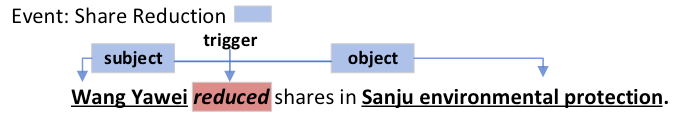• Overlapped Event: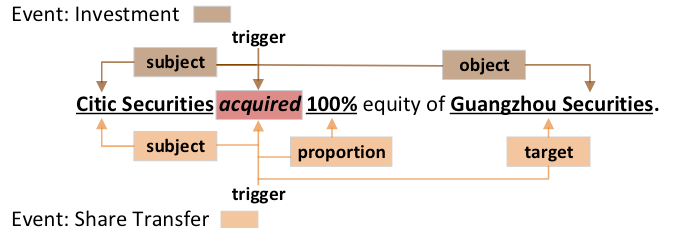重叠事件被定义为拥有相同的触发词或至少拥有一个扮演不同事件角色的相同事件论元事件集.

例如, acquired 同为InvestmentShare Transfer 这两种不同类型的事件的触发词, Guangzhou Securities 在上述两个事件中扮演不同的事件角色.

可能上述表述不够直观, 在CasEE论文中, 将重叠事件区分为三种详细情况:

1. 触发词重叠: 两个不同类型的事件同时共享一个触发词.
2. 多事件论元重叠: 某个论元在多个事件中扮演不同的角色.
3. 单事件论元重叠: 某个论元在同一个事件中扮演不同的角色.

准确的来说, 只要满足上述三种类型其中之一的都可以算作是重叠事件, 满足两条或者三者都满足的也可以是重叠事件.

按照现有的EE论文来看, 相同的论元在不同事件中扮演相同的事件角色是不算重叠的, 至于为什么还不是很清楚.

• Nested Event: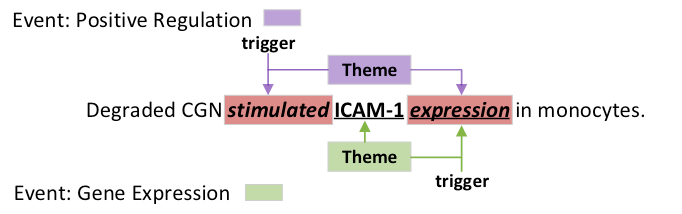嵌套事件被定义为某一事件的论元是另一事件的触发词的两个事件.
例如, 在事件Positive Regulation中, 其Theme 角色的论元expression 是另一事件Gene Expression 的触发词.

## OneEE

### Problem Formulation

• $\mathcal{S}$: Span关系代表起始Token$x_i$ 和结束Token$x_j$ 之间存在的Span关系, S-T, S-A 分别代表触发词的Span和事件论元的Span.
• $\mathcal{R}$: 角色关系意味着触发词$x_i$ 所对应的论元$x_j$ 在事件中扮演的事件角色.
• $\text{NONE}$: 该Word Pair之间不存在任何关系.

• Event: Investment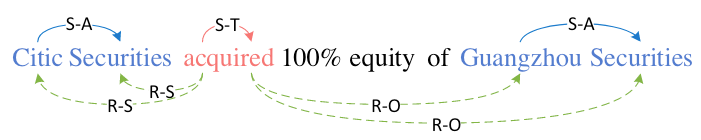在事件类型Investment中, 论元Citic SecuritiesCiticSecurities 之间的Span关系为S-A, 即论元的Span. 同理, 论元Guangzhou SecuritiesGuangzhouSecurities 的Span关系也是S-A. 而该类型对应的触发词acquired 的Span关系为S-T, 代表触发词的Span.

在该类型事件中, 触发词acquired 和论元Citic Securities 中每个Token的角色关系为R-S, 代表Citic Securities 是该事件中的Subject, acquiredGuangzhou Securities 中的每个Token的角色关系为R-O, 代表Guangzhou Securities 是该事件中的Object.

• Event: Transfer Share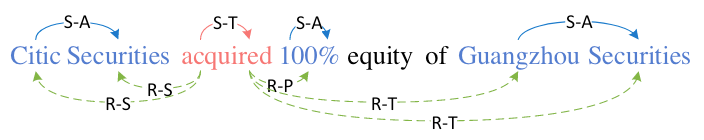还是同样的例子, 在事件类型Transfer Share中, Citic SecuritiesGuangzhou Securities 的Span关系被以同样方式标出. 在角色关系中, 论元Guangzhou Securities 的每个Token和触发词acquired 之间的关系为R-T, 代表Guangzhou Securities 是该事件中的Target. 在该例子中, 100% 也是该事件的论元, 扮演该事件中的Proportion.

### Overview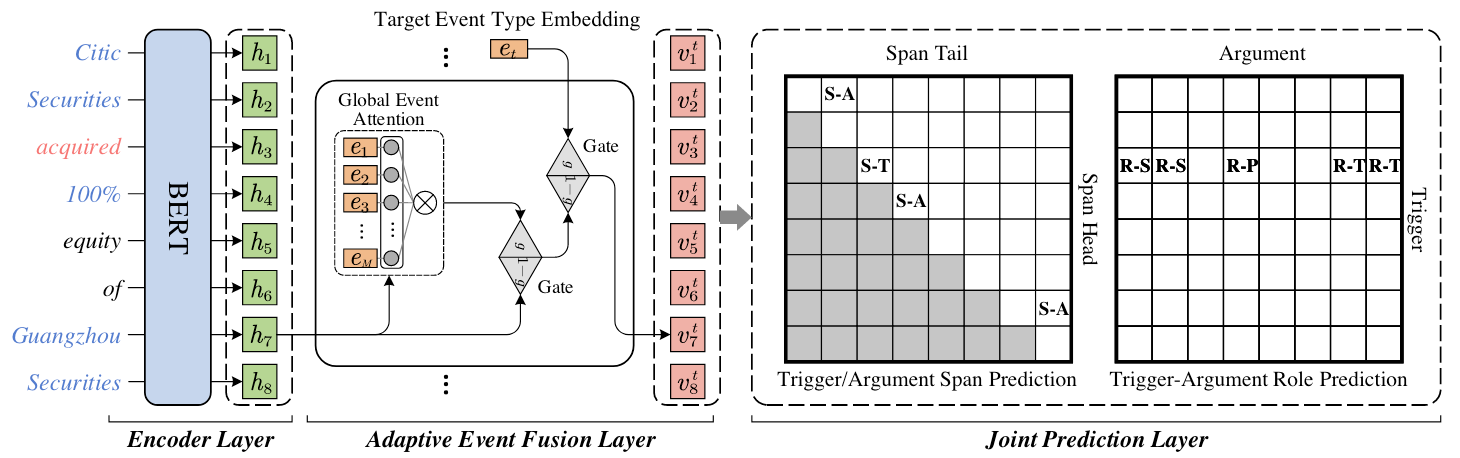#### Encoder Layer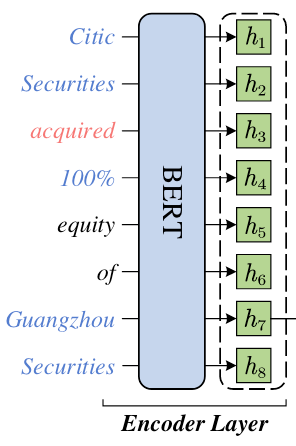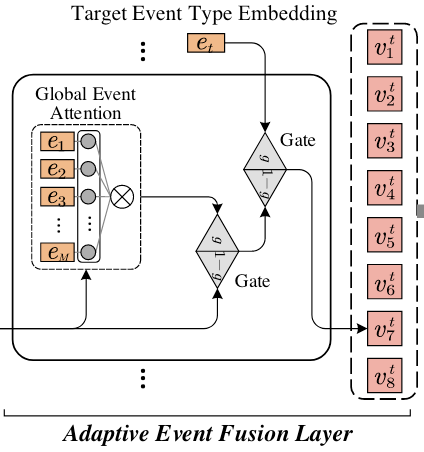##### Attention Mechanism

$$\operatorname{Attention}(\boldsymbol{Q}, \boldsymbol{K}, \boldsymbol{V})=\operatorname{softmax}\left(\frac{\boldsymbol{Q} \boldsymbol{K}^{\top}}{\sqrt{d_h}}\right) \boldsymbol{V}$$

##### Gate Fusion Mechanism

\begin{aligned} \operatorname{Gate}(\boldsymbol{p}, \boldsymbol{q}) &=\boldsymbol{g} \odot \boldsymbol{p}+(1-\boldsymbol{g}) \odot \boldsymbol{q} \\ \boldsymbol{g} &=\sigma\left(\boldsymbol{W}_g[\boldsymbol{p} ; \boldsymbol{q}]+\boldsymbol{b}_g\right) \end{aligned}

$$\boldsymbol{E}^g=\operatorname{Attention}\left(\boldsymbol{W}_q \boldsymbol{H}, \boldsymbol{W}_k \boldsymbol{E}, \boldsymbol{W}_v \boldsymbol{E}\right)$$

\begin{aligned} \boldsymbol{H}^g &=\operatorname{Gate}\left(\boldsymbol{H}, \boldsymbol{E}^g\right) \\ \boldsymbol{V}^t &=\operatorname{Gate}\left(\boldsymbol{H}^g, \boldsymbol{e}_t\right) \end{aligned}

#### Joint Prediction Layer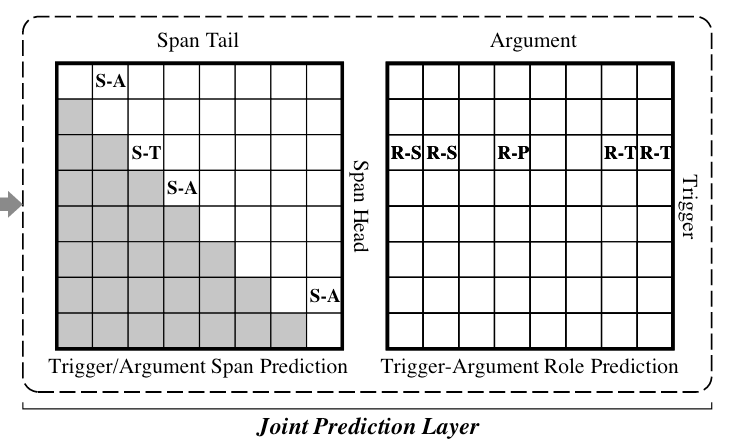#### Distance - Aware Score

\begin{aligned} \operatorname{Score}\left(\boldsymbol{p}_i, \boldsymbol{p}_j\right) &=\left(\boldsymbol{R}_i \boldsymbol{p}_i\right)^{\top}\left(\boldsymbol{R}_j \boldsymbol{p}_j\right) \\ &=\boldsymbol{p}_i^{\top} \boldsymbol{R}_{j-i} \boldsymbol{p}_j \end{aligned}

\begin{aligned} &c_{i j}^s=\operatorname{Score}\left(\boldsymbol{W}_{s 1} \boldsymbol{v}_i^t, \boldsymbol{W}_{s 2} \boldsymbol{v}_j^t\right), \\ &c_{i j}^r=\operatorname{Score}\left(\boldsymbol{W}_{r 1} \boldsymbol{v}_i^t, \boldsymbol{W}_{r 2} \boldsymbol{v}_j^t\right), \end{aligned}

### Training Details

$$\mathcal{L}^{\star}=\log \left(e^\delta+\sum_{(i, j) \in \Omega^{\star}} e^{-c_{i j}^{\star}}\right)+\log \left(e^\delta+\sum_{(i, j) \notin \Omega^{\star}} e^{c_{i j}^{\star}}\right)$$

$$\mathcal{L}=\sum_{t \in \mathcal{E}^{\prime}}\left(\sum_{s \in \mathcal{S}} \mathcal{L}^s+\sum_{r \in \mathcal{R}} \mathcal{L}^r\right)$$

### Inference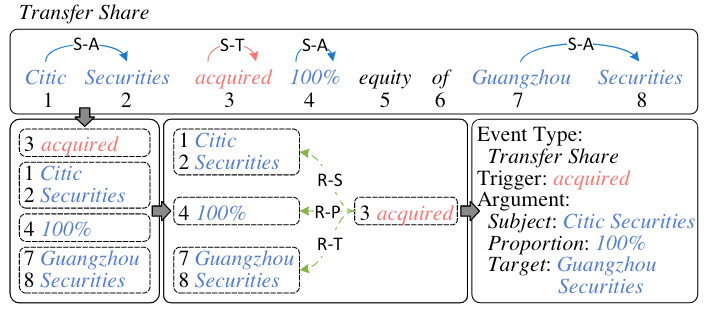1. 找到触发词和论元的起始位置和结束位置.
2. 将对应的起始位置和结束位置整合成触发词或论元的Span.
3. 根据关系$\text{R}-\star$ 匹配论元和触发词的事件角色.
4. 将事件类型赋予该事件.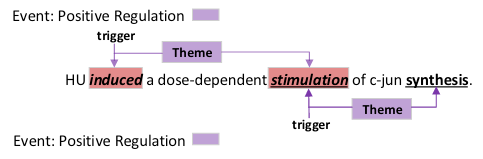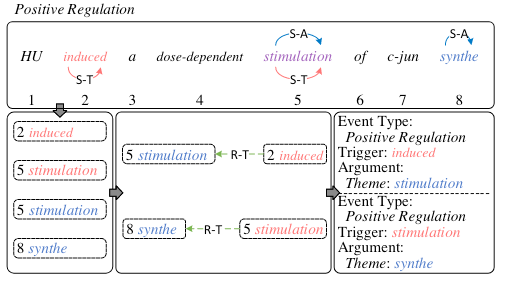## Experiments

### Datasets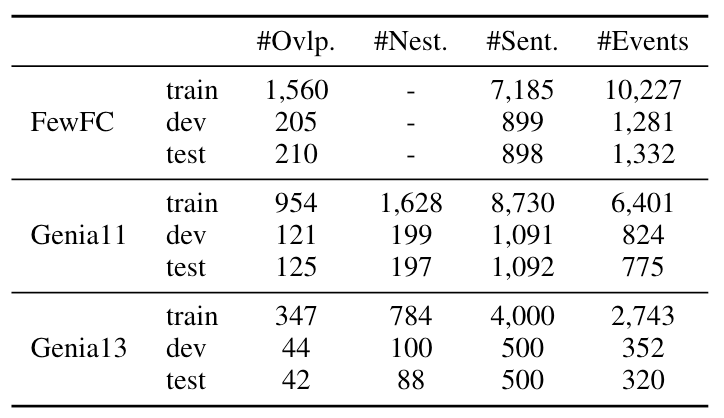### Evaluation Metrics

• Trigger Identification(TI): 触发词Span抽取正确.
• Trigger Classification(TC): 触发词抽取正确, 且触发词所对应的事件分类正确.
• Argument Indentification(AI): 论元Span抽取正确且对应的事件类型正确.
• Argument Classification(AC): 论元抽取正确且其对应的事件角色分类正确.

### All EE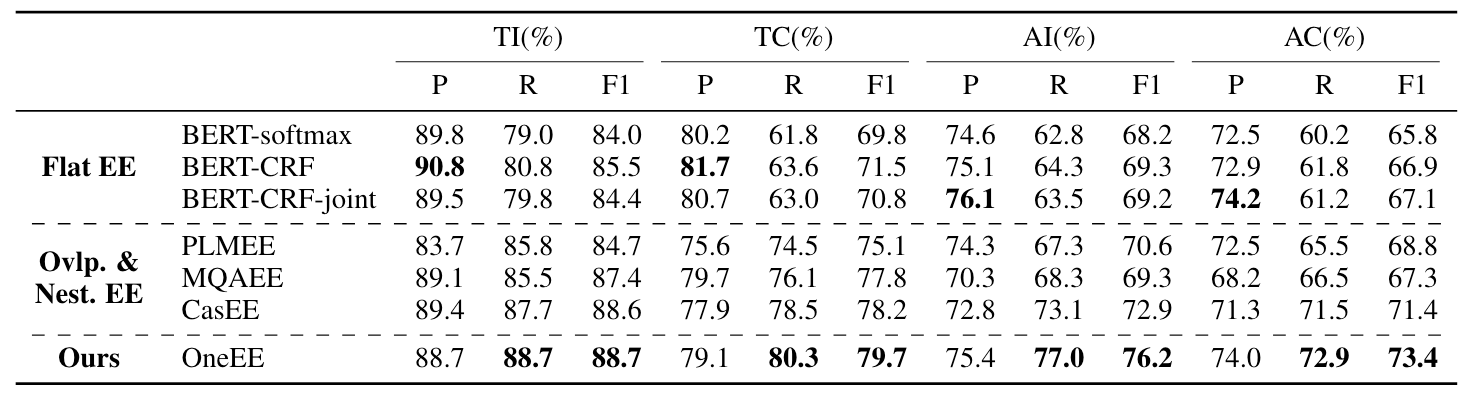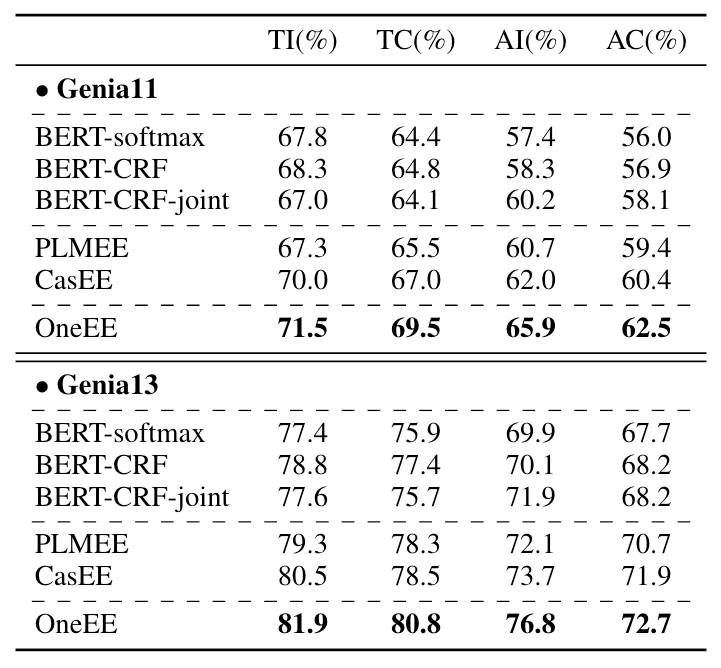### Overlapped and Nested EE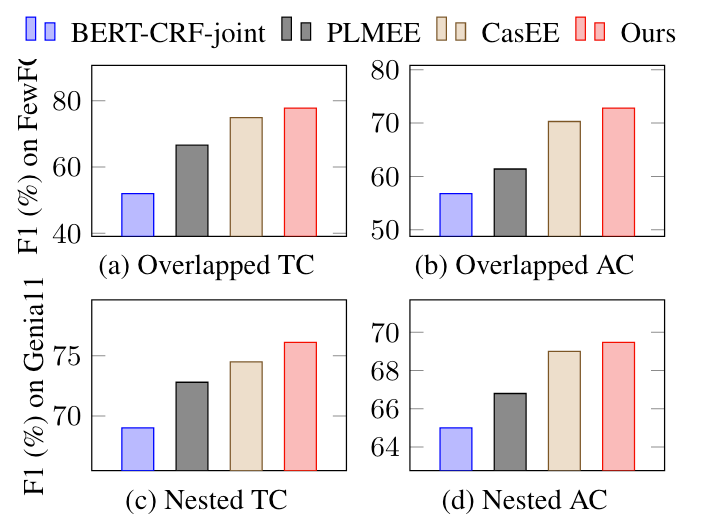(a), (b)图分别代表FewFC中的触发词重叠, 论元重叠. (c), (d)分别代表Genia11中的触发词嵌套和论元嵌套.

### Ablation Study

#### Effects of the Modules in the Fusion Layer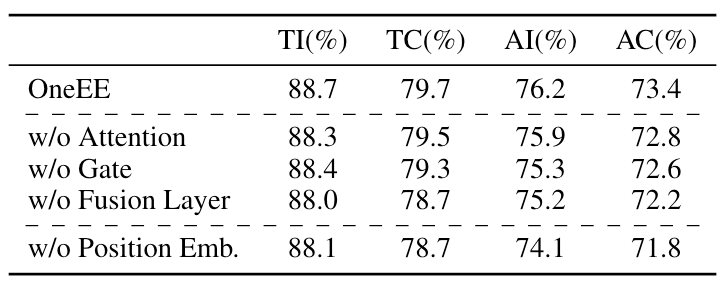w/o Gate 代表作者去掉门控机制, 而更改为将两个向量加在一起, 从结果来看影响并不是很大, 感觉有可能模型能够自己学出来一个过滤掉无用信息的机制?

#### Effect of the Distance - aware Tag Prediction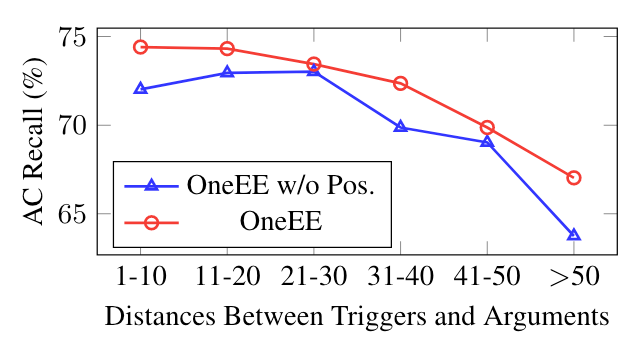### Parameter Number & Efficiency Comparisons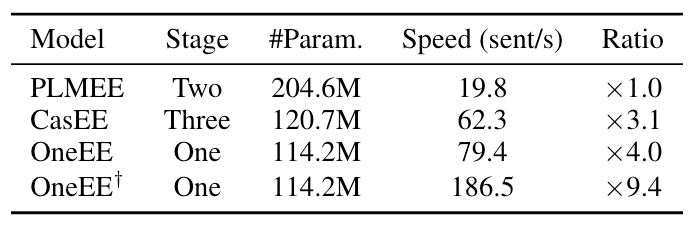### Analysis

#### Analysis of 4 Role Label Strategies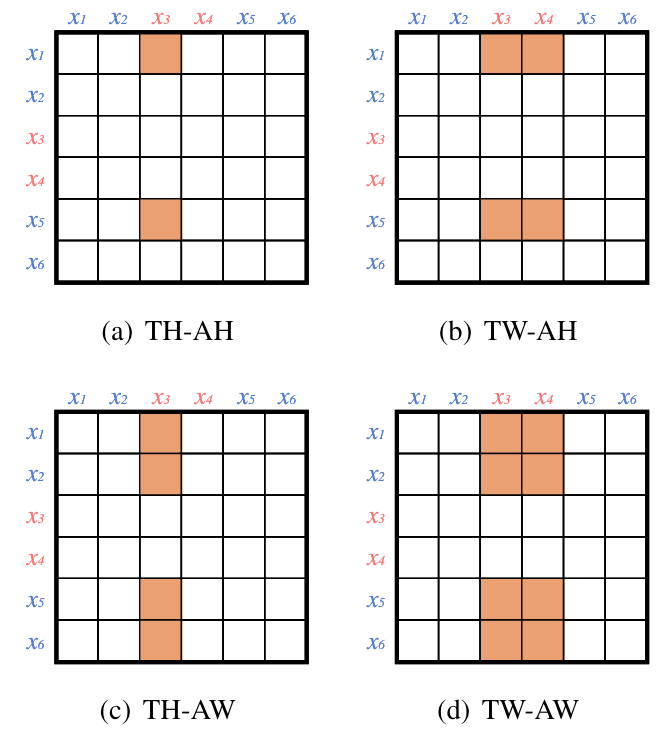• TW-AW(Trigger Word-Argument Word): 标注触发词的所有单词和论元的所有单词.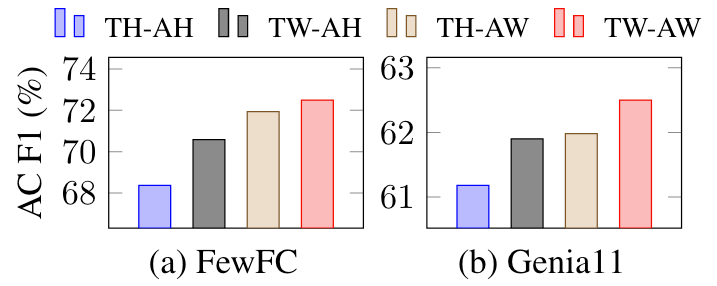TW-AW 相较于其他标注策略要好很多, 作者猜测原因是因为它比其他策略更稠密.

#### Analysis of Event Number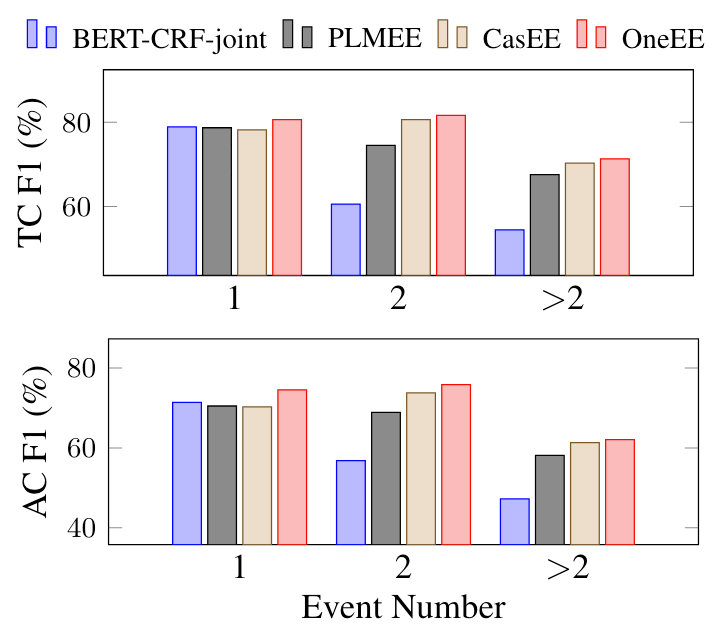## Summary

OneEE采用了一套基于表填充式的单阶段标注策略, 将事件抽取下的Flat Event, Overlapped Event, Nested Event统一词对关系判断问题, 整合到同一个框架下处理, 同时还注意将事件类型作为感知, 融入到表填充过程中, 达到了目前事件抽取领域下的最好结果.

上一篇UniRel: Unified Representation and Interaction for Joint Relational Triple Extraction
UniRel: Unified Representation and Interaction for Joint Relational Triple Extraction本文是论文UniRel: Unified Representation
2023-01-03W2NER: Unified Named Entity Recognition as Word - Word Relation Classification
W2NER: Unified Named Entity Recognition as Word - Word Relation Classification本文是论文Unified Named Entity Recognition as W
2022-10-16
目录